## Bilinear Covariants

We have seen that the constantmatrices can be used to make a conserved vector currentthat transforms correctly under Lorentz transformations. With 4 by 4 matrices, we should be able to make up to 16 components. The vector above represents 4 of those.

The Dirac spinor is transformed by the matrix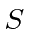.This implies thattransforms according to the equation.Looking at the two transformations, we can write the inverse transformation.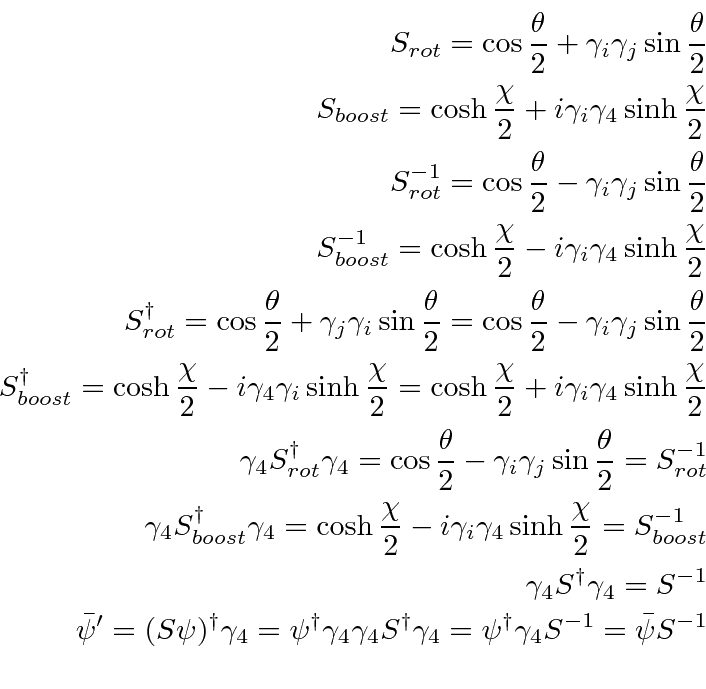This also holds for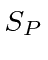.From this we can quickly get thatis invariant under Lorentz transformations and hence is a scalar.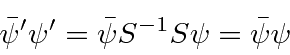Repeating the argument for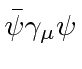we haveaccording to our derivation of the transformations. Under the parity transformation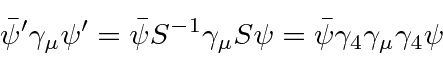the spacial components of the vector change sign and the fourth component doesn't. It transforms like a Lorentz vector under parity.

Similarly, for,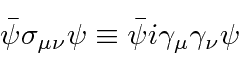forms a rank 2 (antisymmetric) tensor.

We now have 1+4+6 components for the scalar, vector and rank 2 antisymmetric tensor. To get an axial vector and a pseudoscalar, we define the product of all gamma matrices.which obviously anticommutes with all the gamma matrices.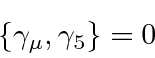For rotations and boosts,commutes withsince it commutes with the pair of gamma matrices. For a parity inversion, it anticommutes with. Therefore its easy to show that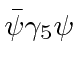transforms like a pseudoscalar and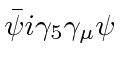transforms like an axial vector. This now brings our total to 16 components of bilinear (in the spinor) covariants. Note that things like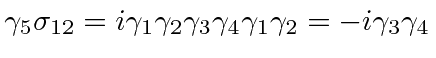is just a constant times another antisymmetric tensor element, so its nothing new.

 Classification Covariant Form no. of Components Scalar1 Pseudoscalar1 Vector4 Axial Vector4 Rank 2 antisymmetric tensor6 Total 16
Thematrices can be used along with Dirac spinors to make a Lorentz scalar, pseudoscalar, vector, axial vector and rank 2 tensor. This is the complete set of covariants, which of course could be used together to make up Lagrangians for physical quantities. All sixteen quantities defined satisfy.

Jim Branson 2013-04-22